Algebra 1 8-8 Complete Lesson: Factoring by Grouping
starstarstarstarstarstarstarstarstarstar
5 (1 rating)
by Matthew Richardson
| 29 Questions
Note from the author:
A complete formative lesson with embedded slideshow, mini lecture screencasts, checks for understanding, practice items, mixed review, and reflection. I create these assignments to supplement each lesson of Pearson's Common Core Edition Algebra 1, Algebra 2, and Geometry courses. See also mathquest.net and twitter.com/mathquestEDU.The outlined content above was added from outside of Formative.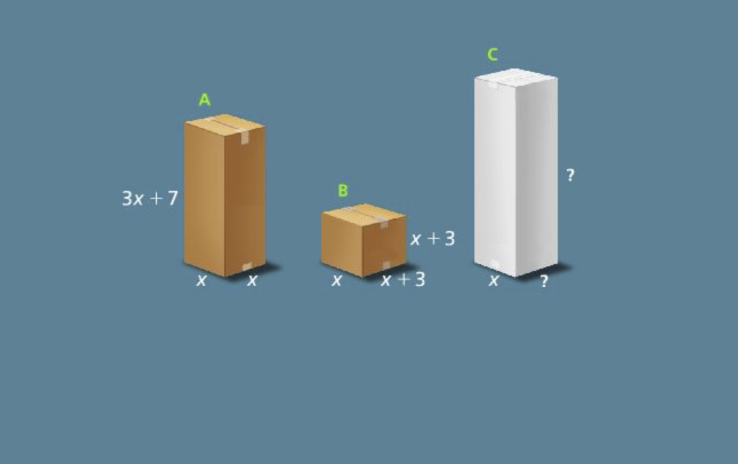1
1
10 pts
Solve It! A packaging company sells two kinds of boxes, Box A and Box B. The company is designing a new box, Box C, that will have the same volume as Boxes A and B combined. Suppose one dimension of Box C is x. What could be the other two dimensions? Select all that apply.
4x + 9
4x + 1
x + 1
x + 9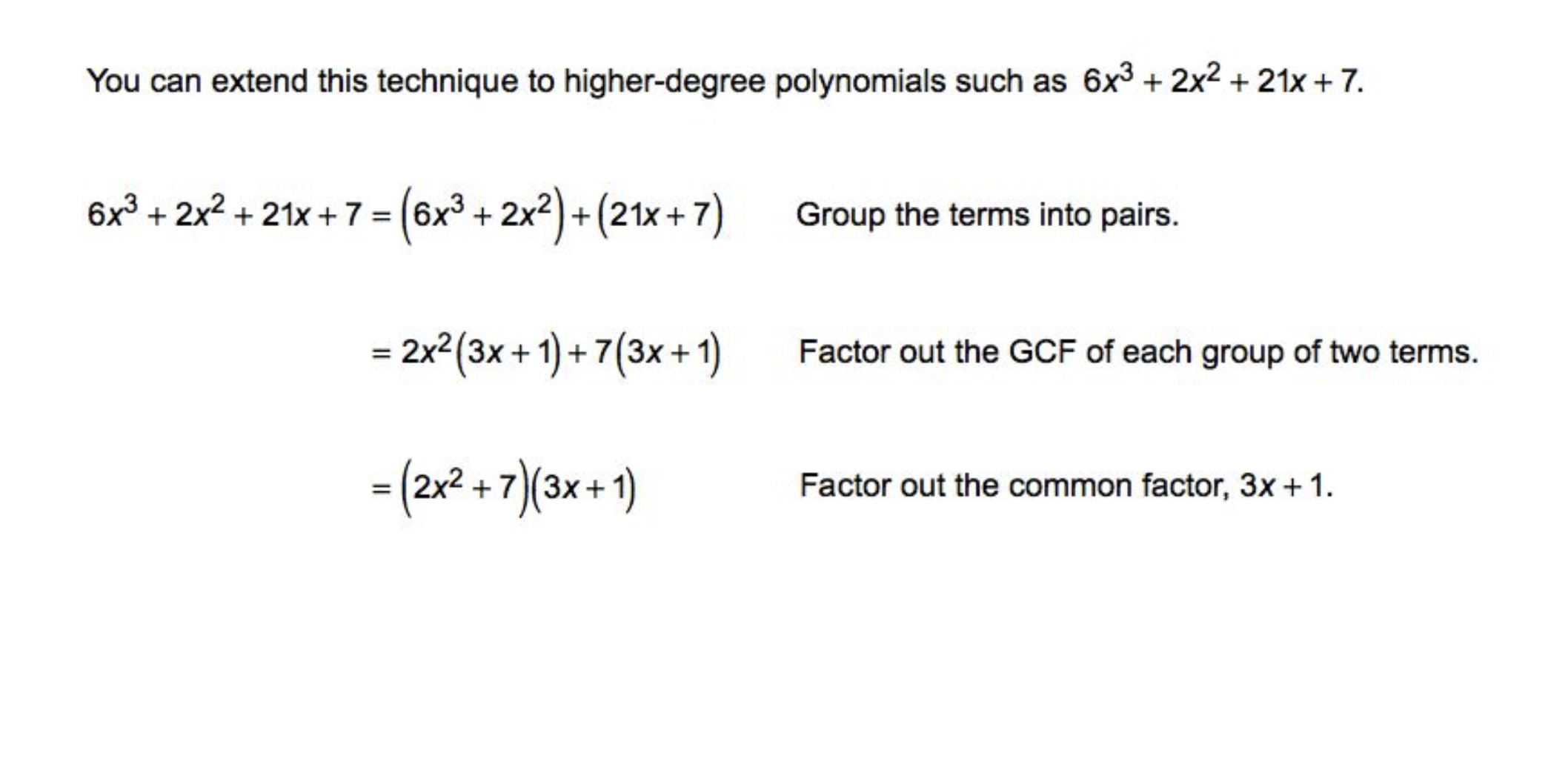2
10 pts
Problem 1 Got It? What is the factored form of the polynomial?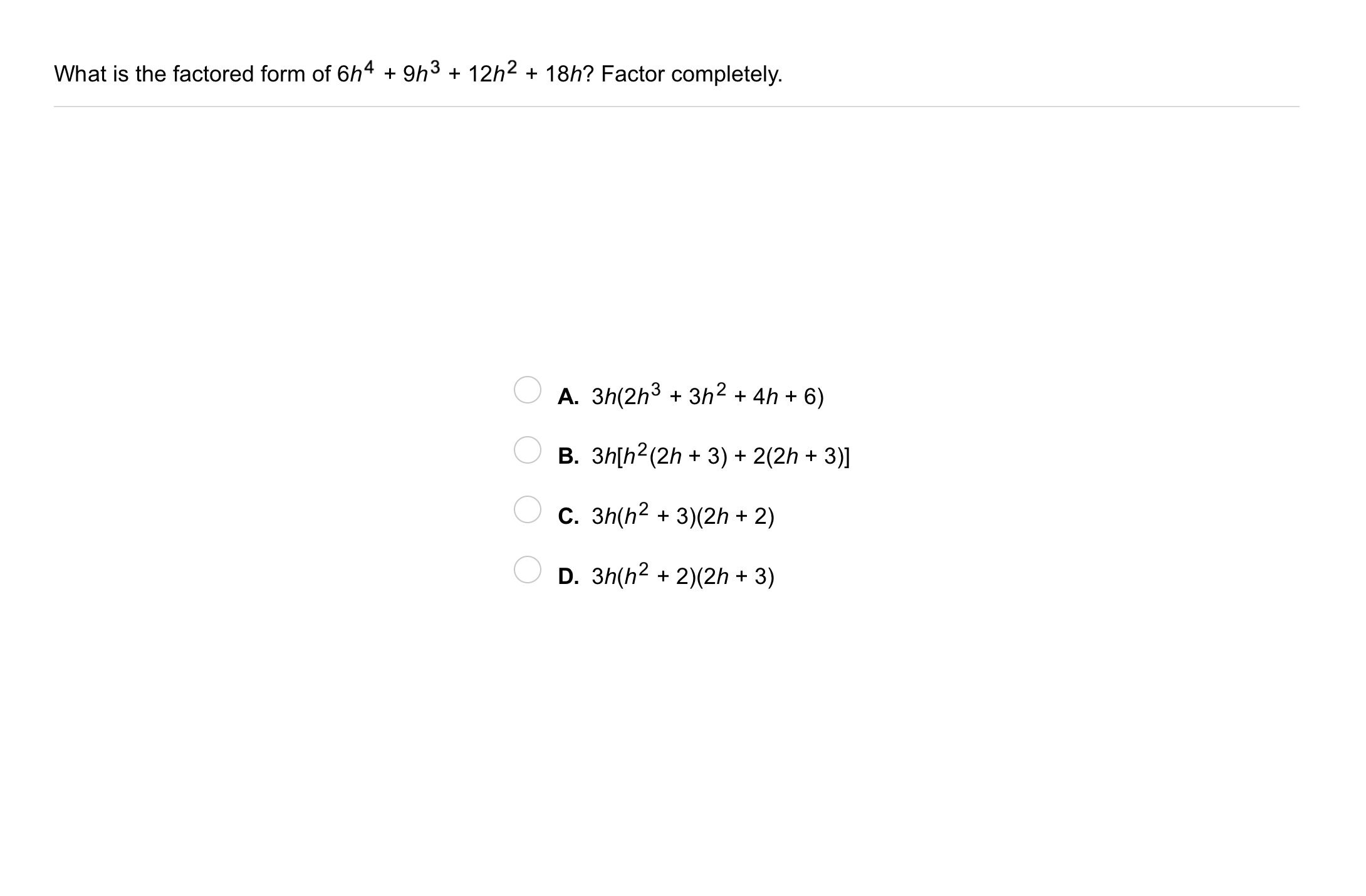3
3
10 pts
Problem 2 Got It?
A
B
C
D
4
10 pts
Problem 3 Got It: Geometry: A rectangular prism has volume represented by the trinomial below.
Which three expressions below could together represent the dimensions of the prism? Use factoring. Select all that apply.
2x
x
6x + 1
5x + 2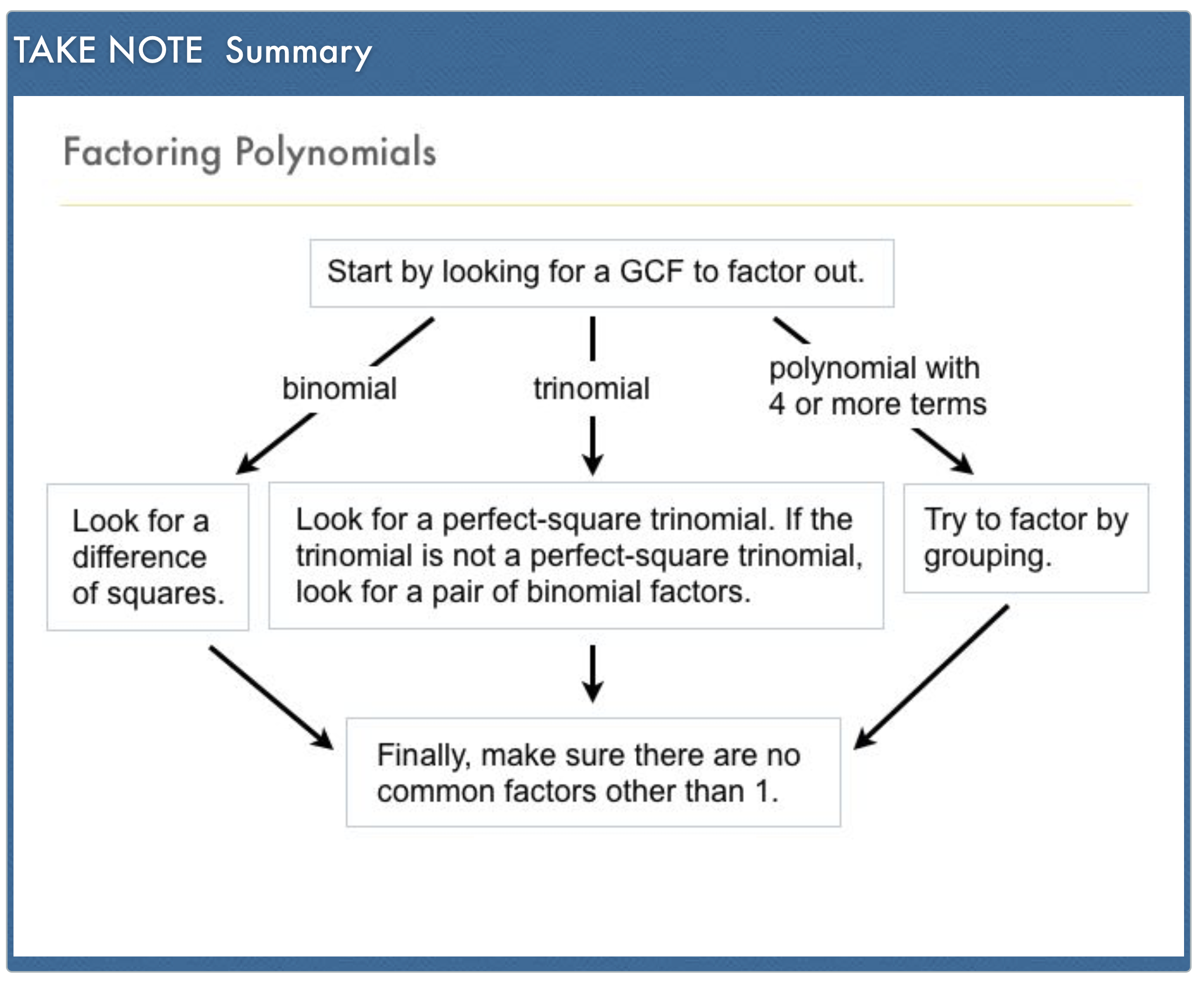5
5
10 pts
A
B
C
D6
6
10 pts
A
B
C
D7
7
10 pts
A
B
C
D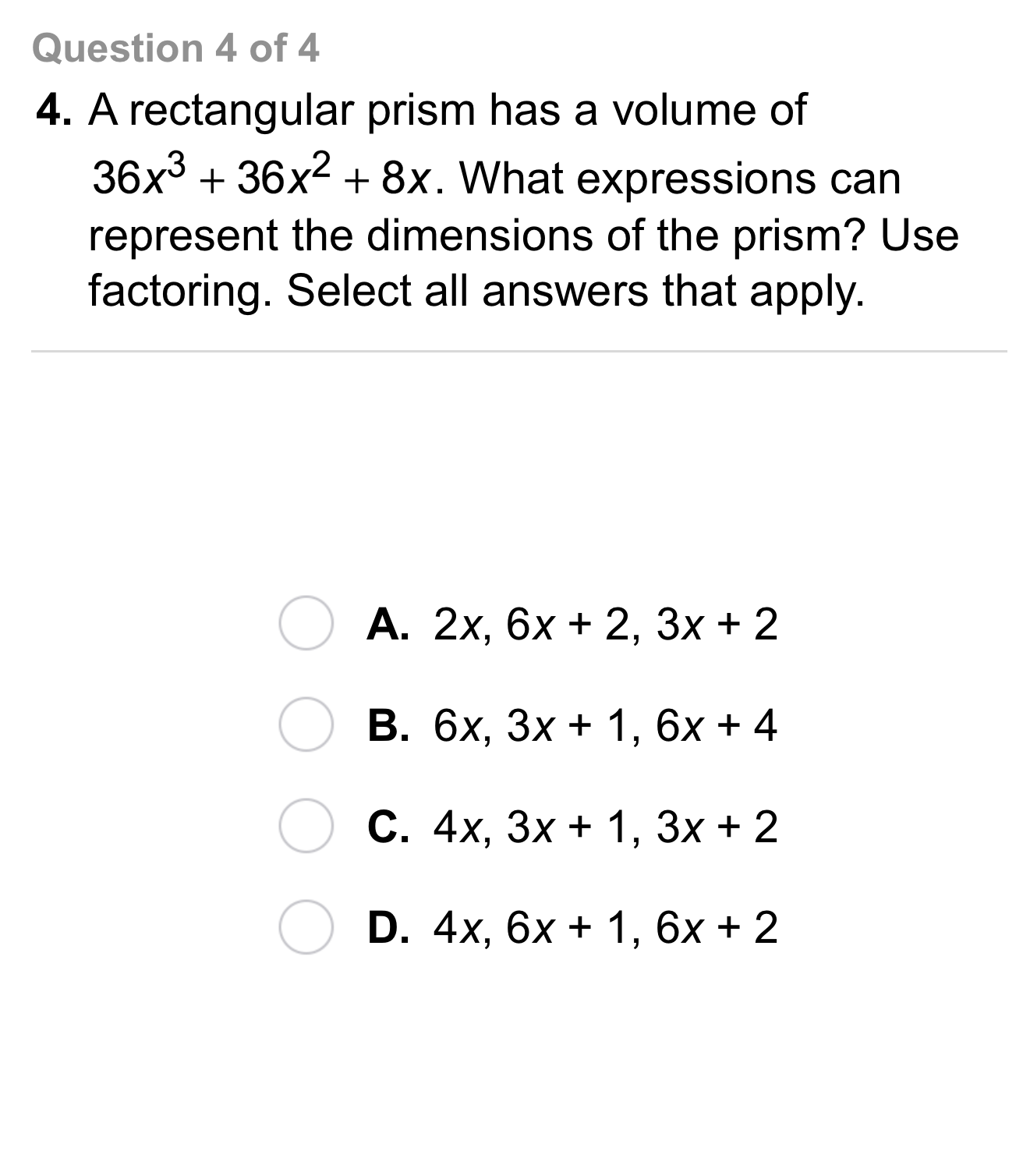8
8
10 pts
Select all that apply.
A
B
C
D9
5 pts
Vocabulary: Tell whether you would factor the polynomial by grouping. Explain your answer in the proceding item.

No, I would not factor by grouping.
Yes, I would factor by grouping.
10
10 pts
Reasoning: Explain your response to the previous item.
11
5 pts
Vocabulary: Tell whether you would factor the polynomial by grouping. Explain your answer in the proceding item.

Yes, I would factor by grouping.
No, I would not factor by grouping.
12
10 pts
Reasoning: Explain your response to the previous item.
13
5 pts
Vocabulary: Tell whether you would factor the polynomial by grouping. Explain your answer in the proceding item.

Yes, I would factor by grouping.
No, I would not factor by grouping.
14
10 pts
Reasoning: Explain your response to the previous item.
15
10 pts
Reasoning: Can you factor the polynomial by grouping? Explain.16
10 pts
Review Lesson 8-7: Factor the expression.
17
10 pts
Review Lesson 8-7: Factor the expression.
18
10 pts
Review Lesson 8-7: Factor the expression.

19
10 pts
Review Lesson 4-6: Use a mapping diagram to determine whether each relation is a function.
• {(4, 3), (3, 4), (4, 7), (7, 4)}
• {(-1, 8), (1, 8), (3, 8), (5, 8)}
• {(2, 7), (4, 7), (6, 7), (8, -7)}
• Function
• Not a function
20
10 pts
Review Lesson 5-3: Use the slope and y-intercept to graph the equation.

21
10 pts
Review Lesson 5-3: Use the slope and y-intercept to graph the equation.

22
10 pts
Review Lesson 5-3: Graph the equation. Zoom and pan your graph to establish an appropriate viewing window.

23
10 pts
Review Lesson 5-3: Graph the equation. Zoom and pan your graph to establish an appropriate viewing window.24
10 pts
Vocabulary Review: Match each expression in the left column with the GCF of its terms in the right column.

• 3x
• 3
• 2x + 1
• 7x²
• 2x26
10 pts
Use Your Vocabulary: Identify each number that is the cube of an integer. Select all that apply.
8
3
12
27
30
27
10 pts
Use Your Vocabulary: Identify the expressions that are NOT cubic polynomials. Select all that apply.

28
100 pts
Notes: Take a clear picture or screenshot of your Cornell notes for this lesson. Upload it to the canvas. Zoom and pan as needed.